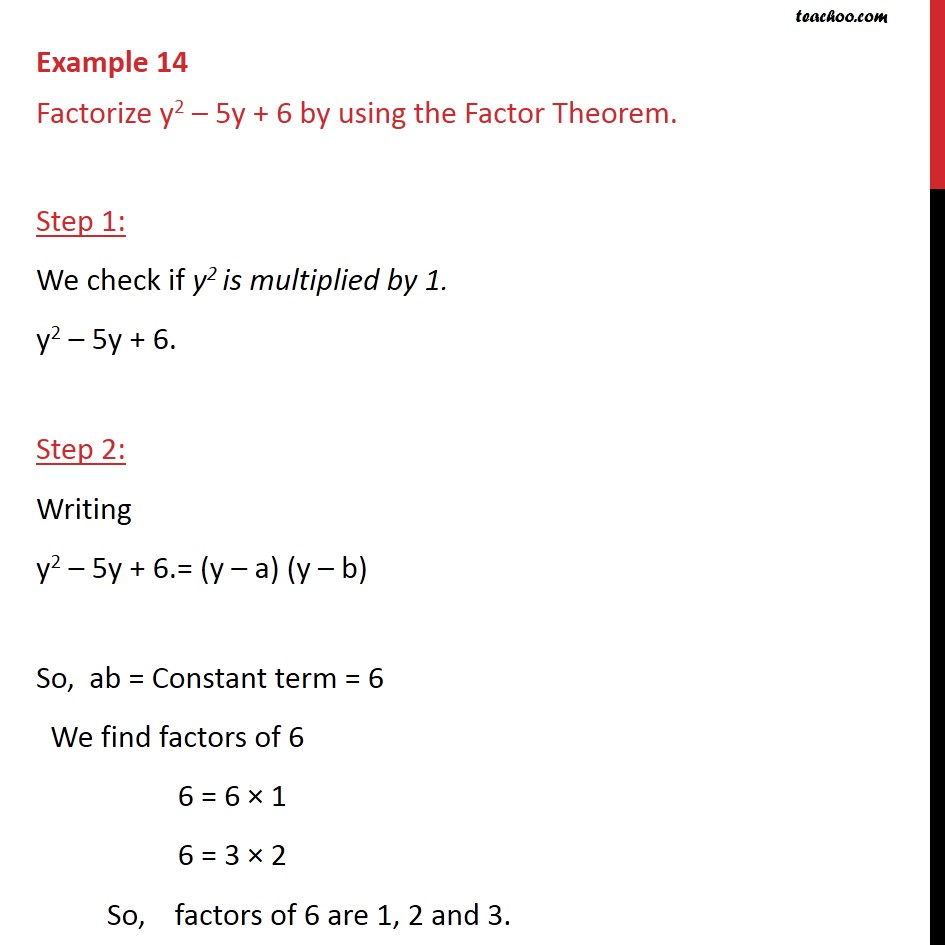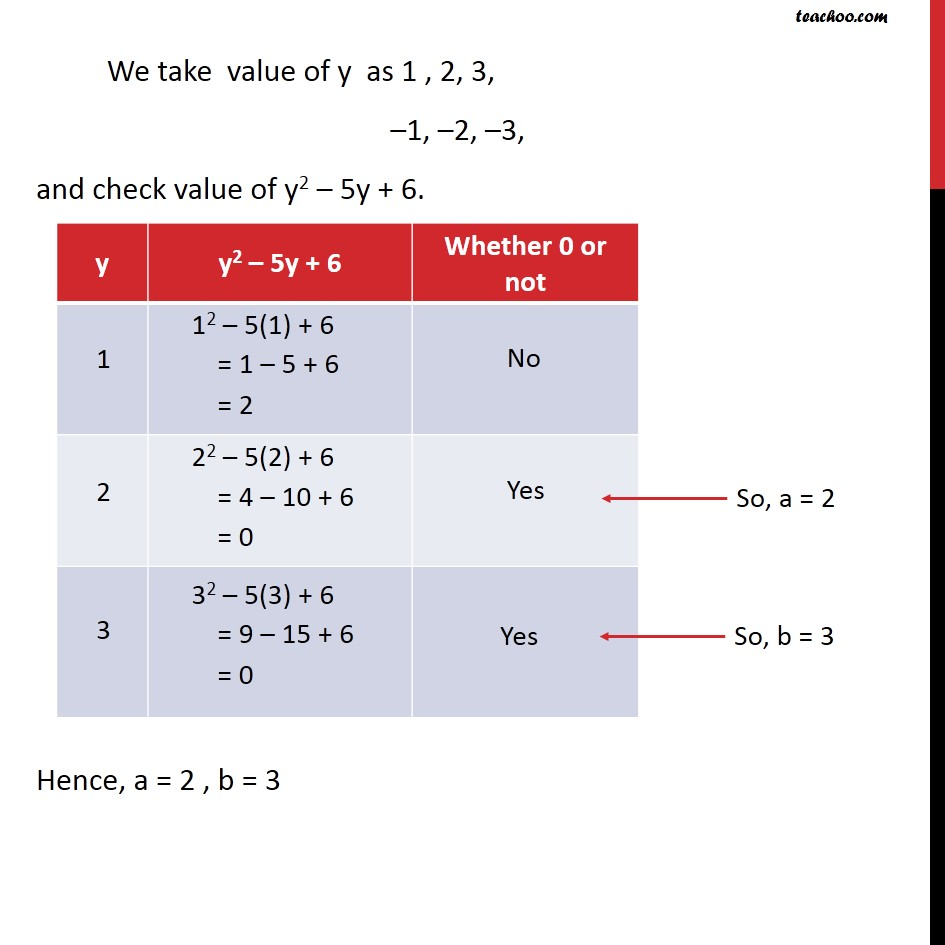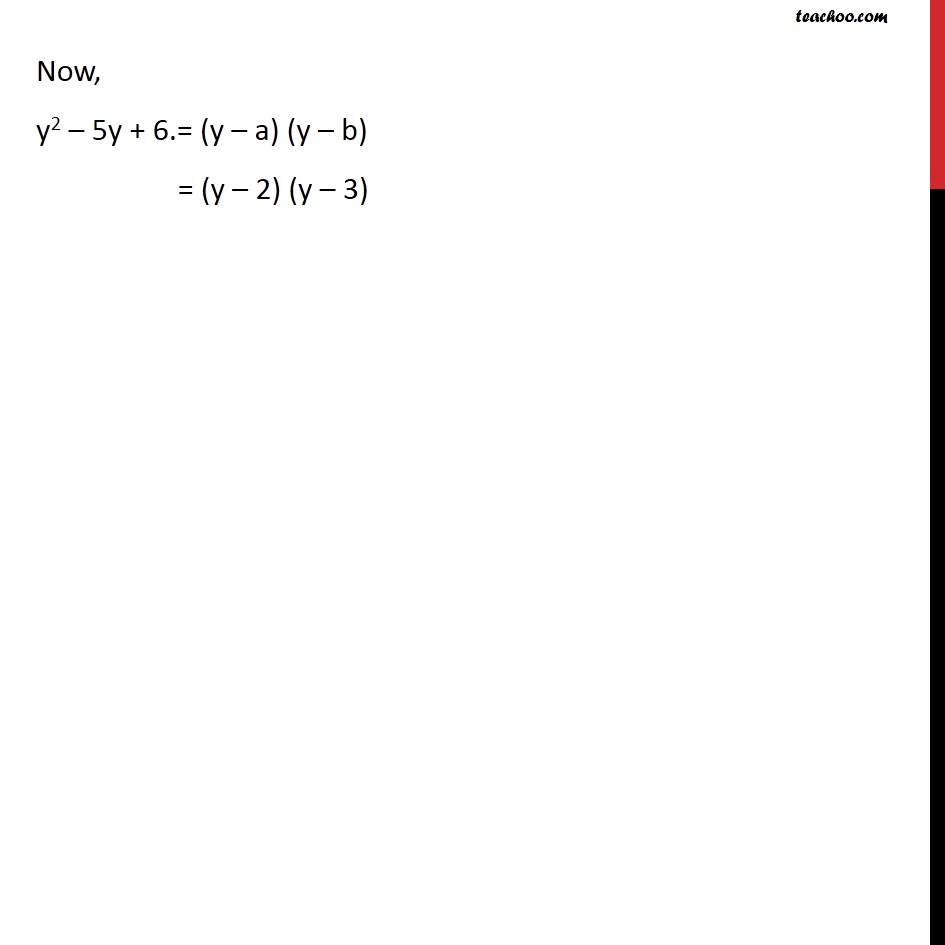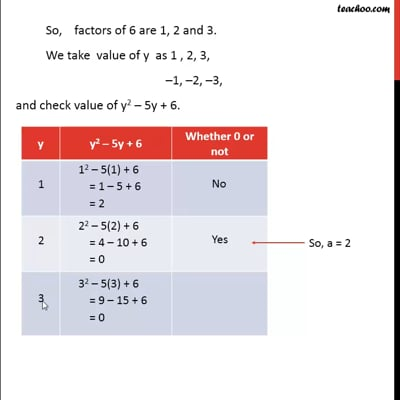Examples

Chapter 2 Class 9 Polynomials
Serial order wiseThis video is only available for Teachoo black users

Solve all your doubts with Teachoo Black (new monthly pack available now!)

### Transcript

Example 14 Factorize y2 – 5y + 6 by using the Factor Theorem. Step 1: We check if y2 is multiplied by 1. y2 – 5y + 6. Step 2: Writing y2 – 5y + 6.= (y – a) (y – b) So, ab = Constant term = 6 We find factors of 6 6 = 6 × 1 6 = 3 × 2 So, factors of 6 are 1, 2 and 3. We take value of y as 1 , 2, 3, –1, –2, –3, and check value of y2 – 5y + 6. Hence, a = 2 , b = 3 Now, y2 – 5y + 6.= (y – a) (y – b) = (y – 2) (y – 3)